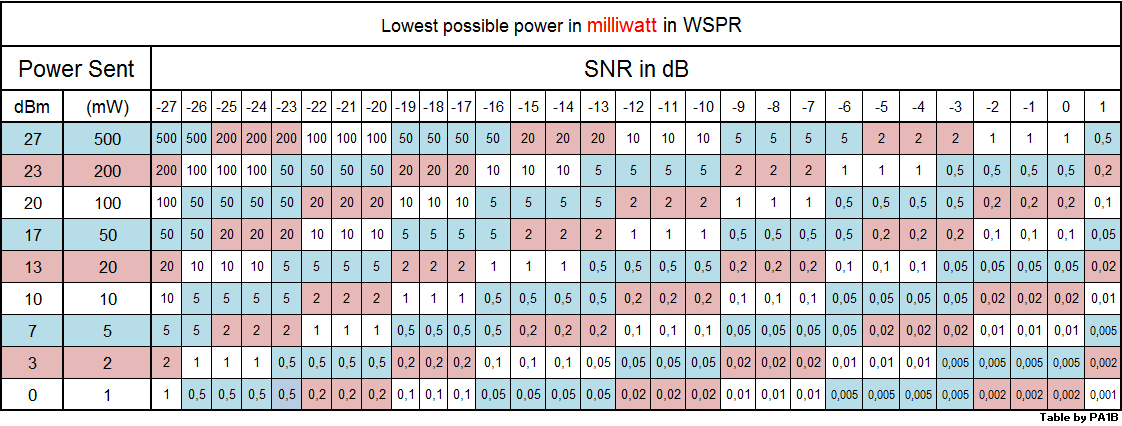### WSPR Propagation Analysis

Changes in propagation give huge changes in signal strength. When the propagation get better, the signal strength and the value of the signal to noise ratio (SNR) will increase.
So the higer the SNR the better the propagation.

To compare WSPR spots, I calculate the minimum power that is required the make a WSPR spot.
When the SNR is -29 dB the WSPR signal will be correctly decoded. So the minimal SNR to calculate the lowest possible power, is -29 dB.

Calculated lowest possible power
A WSPR signal with a SNR of -29 dB is a solid copy. The signal can be received without mistakes.
If the signal has a SNR of -19 dB, the signal is 10 dB stronger than is necessary to be received with an SNR with -29 dB.  So the signal can be reduced with 10 dB. If the power of the transmitting station is 500 mW, the calculated lowest possible power is 50 mW, since the power could be reduced by 10 dB and still be received with a SNR of -29 dB.

The lower the lowest possible power the better the propagation.
The lowest possible power is calculated from the transmitted power and the received SNR.

Determine the lowest possible power with this tableUse this table to determine the lowest possible power of a WSPR spot. PA1B Click to enlarge
Example 1
A WSPR station with a power of 500 mW, is received with a SNR of -9 dB.
Determine the Calculated lowest possible power.

Look at the row of 500 mW. Go to the right, to the column of -9 dB. The value in the field is 5.
The lowest possible power is 5 milliwatt. This means, that if the the station should transmit with 5 milliwatt, the signal would be received with a SNR of -29 dB. A signal with a SNR of -29 dB, is a solid copy in WSPR.

Example 2 (Yellow)
When a signal is received with a SNR of -4 dB and the transmitted power is 200 mW, the lowest possible power is 1 mW. This means that the signal would be received with a SNR of -29 dB when the station would reduce its power to 1 mW.

Example 3 (Green)
In the table below the transmitted power is 100 mW. When the lowest possible power is 20 mW (KL7L) the signal was received with a SNR is -22 dB, -21 dB or -20 dB. (Green)

An analysis with use of the lowest possible powerThe lower the lowest possible power, the better the propagation PA1B
Dave K5MQ made a number of interesting spots on 7 MHz  with 100 mW.
As you can see, not all spots needed a power of 100 mW.
There are many spots that could be made with 10 milliwat or lower. hi.

The lowest possible power gives a great inside in the propagation.

This is a very nice analysis in which you can see the large changes in propagation. For example: in 5 spots Dave needed a power of  100 mW to be received at K9AN. On an other time a power of 0.5 milliwatt should be enough to be received in 6 of the spots. This analysis shows a change of 23 dB in propagation for the signals that are received at K9AN.     10*log (100/0.5) = 23 dB

The calculated lowest possible power, can be used to compare WSPR spots in which the stations  use different powers, in spots are made over the same distance.

The WSPR database (Click to visit) gives the SNR and the used power of each WSPR spot.
You can get much more spots from the database than 50 spot. The maximum number is 20000.